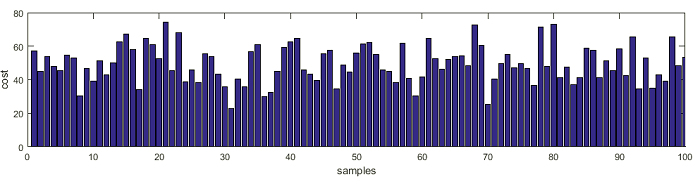## The importance of statistical tests – statistical significance and confidence intervalWhen you start doing more serious research, you will need to conduct a statistical test. It helps you to determine whether your hypothesis is significant or can be rejected. If you start browsing for statistical analyses online, you will find a large number of possible test that can be chosen depending on your task. In this post, let us focus on a simple example of statistical analysis that would allow us to understand what is statistical significance and what is confidence interval. Building a test scenario To understand the matter lets use some random data which is distributed normally. For this, we can use 100 casual observations of the price tag for the new Nokia 3310 from different sources. The average price is around 50€ with a standard deviation of 10€ Now lets plot how those samples are distributed along with the standard distribution graph: